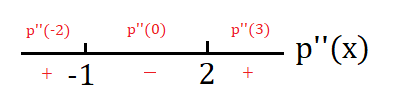# Let p be a function whose second derivative is p"(x) = (x + l)(x - 2)e^-x . (a) Construct a...

## Question:

Let p be a function whose second derivative is p"(x) = {eq}(x + l)(x - 2)e^-x {/eq}.

(a) Construct a second derivative sign chart for p and determine all inflection points of p.

(b) Suppose you also know that x = {eq}\sqrt 5-1/2 {/eq} is a critical number of p. Does p have a local minimum, local maximum, or neither at x = {eq}\sqrt 5-1/2 {/eq}? Why?

(c) If the point {eq}(2,12/e^2) {/eq} lies on the graph of y = p(x) and p'(2) = {eq}-5/e^2 {/eq}, find the equation of the tangent line to y = p(x) at the point where x = 2. Does the tangent line lie above the curve, below the curve, or neither at this value? Why?

## Inflection Points of graphs

An inflection point of a graph of a function {eq}f(x) {/eq} is a point at which the function either changes from being concave up to concave down or from being concave down to concave up. To find any possible point of inflection, find where the second derivative {eq}f''(x) {/eq} is equal to zero or undefined. After finding all possible points of inflection, check the concavity of the function on each side of the possible points by substituting values on each side of the possible point into the second derivative - if the value is positive, the graph is concave up and if the value is negative, the graph is concave down. If the function does indeed change concavity at the point, then you have an inflection point.

Note that the function's second derivative should read as {eq}p''(x)=(x+1)(x-2)e^{-x} {/eq}

a) The possible inflection points are where the second derivative is undefined or equal to zero. The second derivative is never undefined and it equals zero when {eq}x=-1,2 {/eq}

Checking values on either side of each possible point of inflection, we see that both possible points of inflection are inflection points.b) Since {eq}x=\sqrt{5}-\frac{1}{2}\approx 1.74 {/eq}, we see on the sign chart that {eq}p''\left(\sqrt{5}-\frac{1}{2}\right)<0 {/eq}, so by the second derivative test, we have a local maximum at {eq}x=\sqrt{5}-\frac{1}{2} {/eq}

c) Since the derivative provides the slope of the tangent line, the equation of the tangent line at this point is

{eq}y=\frac{12}{e^2}-\frac{5}{e^2}(x-2)\\ y=-\frac{5}{e^2}+\frac{22}{e^2} {/eq}

The tangent line does not lie above or below at this value since a tangent line lies below the curve if the curve is concave up and it lies above the curve if the curve is concave down - but {eq}x=2 {/eq} is an inflection point - where the graph changes concavity.